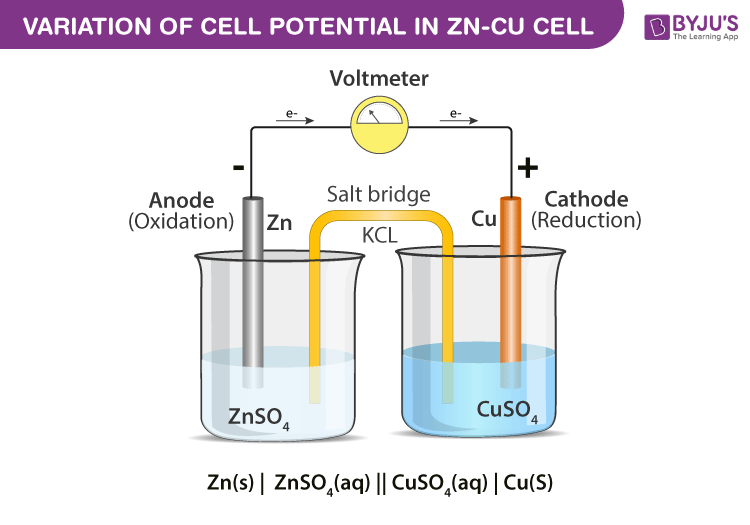# Explain Daniel Cell With Cell Diagram Representation And Process Taking Place In The Cell

The electrochemical cell, which converts chemical energy into electrical energy, is called a Daniell cell.

The Daniell cell consists of two electrodes of dissimilar metals, Zn and Cu

each electrode is in contact with a solution of its ion; Zinc sulphate and copper sulphate respectively.

A Daniell cell is the best example of a galvanic cell.

Daniel Cell DiagramCell reaction at Anode is

$$Zn_{(s)}\rightarrow Zn_{(aq)}^{2+} + 2e^{-}$$ (Oxidation)

Cell reaction at Cathode is

$$Cu_{(aq)}^{2+} + 2e^{-}\rightarrow Cu_{(s)}$$ (Reduction)

Overall, Cell reaction is

$$Zn_{(s)} + Cu_{(aq)}^{2+} \rightarrow Zn_{(aq)}^{2+} + Cu_{(s)}$$

Explore more such questions and answers at BYJU’S.